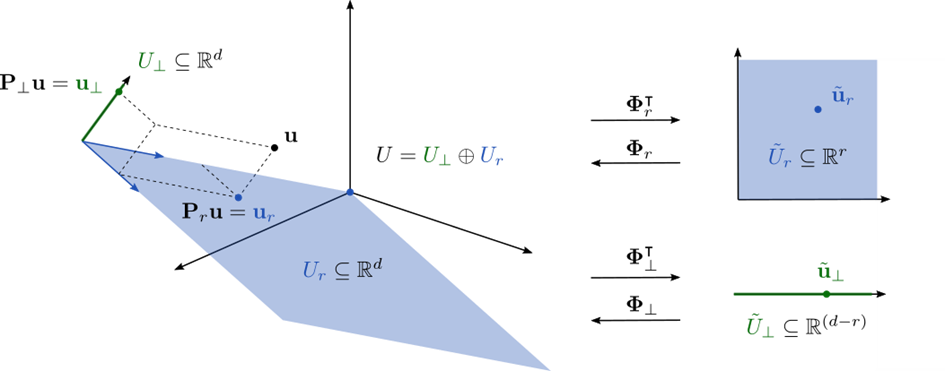# Dimensionality Challenges in UQ for Inverse Problems

Rafael Flock: Shrinking large dimensional Bayesian inverse problems

Inverse problems arise in many areas of applied science such as for example engineering applications. Generally, in these problems we try to infer the true state of the world through measurements. However, we often cannot directly measure the parameters we are looking for, i.e., the parameters that represent the true state of reality. Then we need models that can compute measurable quantities for a set of given parameters. Based on the measurements from reality, we can then try to infer the parameters using the model. That is, instead of using the model in the forward direction, i.e., plugging into it the parameters and calculating the measurable quantities, we use the model in the reverse direction, i.e., we infer the parameters of the model using the measurable quantities. This kind of problem is called an inverse problem.

For example, consider the task of determining the condition of the concrete of the girders of a simply supported bridge. We might have a computational model of the bridge that, given the material strength of the girders, allows us to compute the vertical deformations at various locations on the bridge. Now to determine the material strength, we cannot simply drill holes in the girders to take samples as this would compromise the stability of the bridge. However, we could take measurements of the vertical deformation at various locations along the bridge. Together with our computational model, we can then use the measurements for the inference of the parameters of the model, i.e., the material strength of the bridge.

Since measurements are usually not exact and computational models can never perfectly represent reality, we need to quantify the uncertainty of our results. For example, we try to obtain a probability distribution of the inferred parameters, or at least some statistical descriptors such as the standard deviation or credible intervals. We can do this by solving the problem in a Bayesian framework. A key challenge in solving Bayesian inverse problems is the number of parameters involved, i.e., the dimensionality of the problem. The larger this number the more time consuming it is to compute a solution. To illustrate the challenges involved, consider again the bridge example.

Imagine that the bridge is 100 m long, and we want to know the strength of the material at two points every 10 cm. For this task, we would end up with 2000 unknown parameters. For many other applications, this number is even larger. Consider also the case where we have repeated measurements available. In this case, we would need to update our high-dimensional model every time we get a new set of data. Furthermore, it might be necessary that this task does not take too much time since the results must be available immediately.

In this PhD project, we study different types of dimensionality challenges in Bayesian inverse problems. The goal is to develop computationally efficient methods tailored to the different types of problems. In general, a promising approach is to project the high-dimensional parameter space onto a low-dimensional parameter space. The figure above/below illustrates this procedure for an example parameter space of dimension three. In the full parameter space on the left, the blue plane represents the subspace to which we reduce the parameter space. We can use the available data and our forward model to define this space. By restricting the unknown parameters to this plane, we reduce the dimensionality of the problem from three to two. For real-world applications, of course, we aim for a much larger difference, such as reducing the dimension from, e.g., 1 million to 500. Finally, the inverse problem can be solved more efficiently because much fewer parameters are involved. In the end, the solution of the reduced problem is projected back to the full-dimensional space.

This project is part of the CUQI (Computational Uncertainty Quantification for Inverse problems) research initiative funded by Villum Fonden (https://www.compute.dtu.dk/english/cuqi). The goal of CUQI is to make uncertainty quantification (UQ) for inverse problems easily accessible to non-experts.

## PhD project

By: Rafael Flock

Section: Scientific Computing

Principal supervisor: Yiqiu Dong

Co-supervisor: Olivier Zahm (INRIA)

Project title: Dimensionality Challenges in UQ for Inverse Problems

Term: 01/10/2021 → 30/09/2024

## ContactRafael Flock
PhD student
DTU Compute

## ContactYiqiu Dong
Associate Professor
DTU Compute
+45 45 25 31 08
https://www.compute.dtu.dk/english/phd/current-phd/phd-sc/rafael-flock
10 JUNE 2023